Calculator Regression Models

A mathematical model is an equation that best describes a particular set of paired data.
The mathematical models shown here are referred to as
regression models and are used to predict one variable based upon another variable.

The linear based regression models on the graphing calculator:

 • Linear (LinReg) y = ax + b The graph of x versus y is linear. Fits Linear by Transformations: • Logarithmic (LnReg) y = a + b ln(x) The graph of ln(x) versus y is linear.   Calculates a and b using linear least squares on lists of ln(x) and y instead of x and y. • Exponential (ExpReg) y = a (b x) The graph of x versus ln(y) is linear.Calculates A and B using linear least squares on lists of x and ln(y) instead of x and y, and then a = eA and b = eB . • Power (PwrReg) y = a ( x b) The graph of ln(x) versus ln(y) is linear.Calculates A and b using liner least squares on list of ln(x) and ln(y) instead of x and y, and then a = eA.

Other models available on the graphing calculator:

 • Quadratic (QuadReg)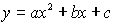For three points, fits a polynomial to the data.  For more than three points, fits a polynomial regression. • Cubic (CubicReg)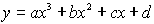For four points, fits a polynomial to the data.  For more than four points, fits a polynomial regression. • Quartic (QuartReg)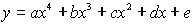For five points, fits a polynomial to the data.  For more than five points, fits a polynomial regression. • Logistic (Logistic)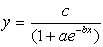Fits equation to data using iterative least-squares fit. • Sinusoidal (SinReg)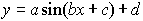Fits sine wave to data using iterative least-squares fit.Finding Your Way Around TABLE of  CONTENTS